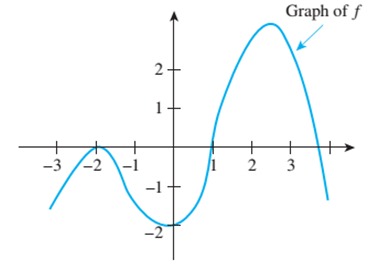Chapter 11.1, Problem 14ES### Discrete Mathematics With Applicat...

5th Edition
EPP + 1 other
ISBN: 9781337694193

#### Solutions

Chapter
Section### Discrete Mathematics With Applicat...

5th Edition
EPP + 1 other
ISBN: 9781337694193
Textbook Problem
1 views

# The graph of a function f is shown below. Find the intervals on which f is increasing and the intervals on which f is decreasing.To determine

Find the intervals on which f is increasing and the intervals on which f is decreasing.

Explanation

Given information:

Concept used:

Function is increasing if the slope is positive and in case of decreasing, function is decreasing.

Calculation:

f Is increasing on the intervals

{xR|3<x<2}

And {xR|0<x<2

### Still sussing out bartleby?

Check out a sample textbook solution.

See a sample solution

#### The Solution to Your Study Problems

Bartleby provides explanations to thousands of textbook problems written by our experts, many with advanced degrees!

Get Started